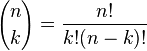## Pascal’s triangle

Numerology is an old phenomenon in many cultures. It is represented both in art an buildings. Buildings are constructed according tothe Golden Section and magical quadrats can be found in eg Albrect Durer’s painting ”Melancholy”.

A substantial amount of mathematics is hidden in Pascal’s triangle. This triangle isbased on the principle that an element in it is the sum of the nearest elements above it.The elements in Pascal’s triangle turns out to be the binomial-coefficients. This means that they are identical to the numbers you get when you expand binomials with different exponentials. A binomial is the sum of two numbers (x + y) .

As an example we can take (x+y)^2 = x^2 + 2xy + y^2.

The coefficients here can be found in the second row of the periodic system: 1    2    1.

The  coefficients for (x + y) ^3 is given by the third row: 1  3  3   1 and so on.

This is spectacular in its own right but it doesn’t   end there: The binomialcoefficients also have a combinatorical interpretation: They give the  number of combinations if you e.g. are to select k objects out of n possible.

Example: Suppose you wish to pick 2  apples out of a set of three. You find the answer as the second element in the third row: 3.

This is called the number of combinations possible when selecting two elements from a set of three. In a combination the order is irrelevant.   According to convention it is writen asHere n! = the number of permutations of n i.e. the number of possible arrangements of n objects when the order is important, k! is the number of permutations of k objects and (n-k)! the number of permutations of the difference of n and k.
Annons## Om mattelararen

Licentiate of Philosophy in atomic Physics Master of Science in Physics
Detta inlägg publicerades i Uncategorized och märktes , . Bokmärk permalänken.

### 2 kommentarer till Pascal’s triangle

1.Pelle skriver:

Vad bra att man kan få hjälp här! Killen längst bak sitter inne på andra websidor

•mattelararen skriver:

Du är välkommen med matte och fysik-frågor.